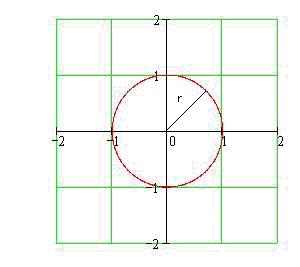Calculus on Demand at Dartmouth College Lecture 27 | Index | Lecture 29 Lecture 28## Resources

Math 3 Course Home Page
Math 3 Course Syllabus
Practice Exams
Textbook Home Page
Post a Comment

# Contents

In this lecture we solve word problems involving variables that are linked through an equation that relates their rates of change.

### Quick Question

If the radius of a circle is increasing at a rate of 2 m/s, how fast is the area growing when the radius equals 4 meters?Answer

Related Rates

Log into WebWorK

### Quiz

Related Rates Quiz

### Examples

•Suppose that an inflating balloon is spherical in shape, and its radius is changing at the rate of 3 centimeters per second. At what rate is the volume changing when the radius is 10 centimeters?
•A baseball diamond is 90 feet square, and the pitcher's mound is at the center of the square. If a pitcher throws a baseball at 100 miles per hour, how fast is the distance between the ball and first base changing as the ball crosses home plate?
•A ladder 10 feet long is resting against a wall. If the bottom of the ladder is sliding away from the wall at a rate of 1 foot per second, how fast is the top of the ladder moving down when the bottom of the ladder is 8 feet from the wall?
there are no videos for this section

### Videos

Lecture 27 | Index | Lecture 29